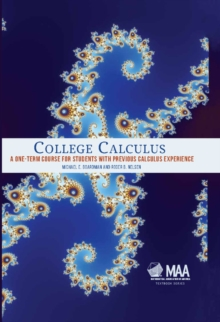Supporting your high street Find out how »
• My Account# College Calculus : A One-Term Course for Students with Previous Calculus Experience Hardback

#### Description

For students who have completed an introductory calculus course in high school, this textbook provides a thorough grounding in many subsequent single variable calculus topics.

Beginning with a review of some high school calculus content, it proceeds to more advanced material including integration techniques, applications of the definite integral, separable and linear differential equations, hyperbolic functions, parametric equations and polar coordinates, L'Hopital's rule and improper integrals, continuous probability models, and infinite series.

Each chapter concludes with several 'Explorations', extended discovery investigations to supplement that chapter's material and enhance the learning experience.

The text is ideal as the basis of a course for prospective majors in the STEM disciplines (science, technology, engineering, and mathematics).

A one-term course based on this text provides students with a solid foundation in single variable calculus and prepares them for the next course in college-level mathematics, be it multivariable calculus, linear algebra, discrete mathematics or statistics.

#### Information

• Format: Hardback
• Pages: 383 pages
• Publisher: Mathematical Association of America
• Publication Date:
• Category: Calculus & mathematical analysis
• ISBN: 9781939512062

£40.99

£37.19£45.00

£37.59